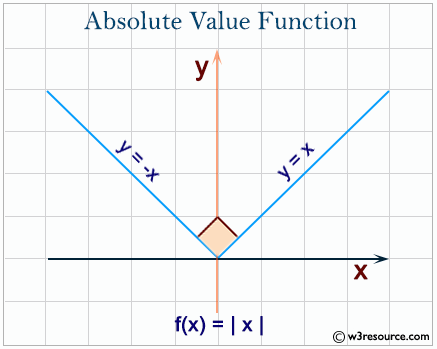# Python: abs() function

## abs() function

The abs() function is used to get the absolute (positive) value of a given number.

The argument may be an integer or a floating point number. If the argument is a complex number, its magnitude is returned.

Syntax:

abs(x)Version:

(Python 3)

Parameter:

Name Description Required / Optional
number Numeric value. Required

If x is a complex number function returns it's magnitude. The absolute value  of a complex number z = x + yj isReturn value:

Return the absolute value of a number.

Pictorial Presentation:Example: Python abs() function

print(abs(-100))
print(abs(1023))
print(abs(123.25))
print(abs(-2033.66))


Output:

100
1023
123.25
2033.66


In Python abs() works with integers and complex numbers. It's return type depends on the type of its argument.

>>> type(-2)
<class 'int'>
>>> type(abs(-2))
<class 'int'>
>>> type(-2.0)
<class 'float'>
>>> type(3+4j)
<class 'complex'>
>>> type(abs(3+4j))
<class 'float'>
>>>


Get absolute value of numbers of a list:

nums = [12,30,-33,-22,77,-100]
print("Original list:")
print(nums)
print("\nAbsolute values of the above numbers:")
print([abs(x) for x in nums])


Output:

Original list:
[12, 30, -33, -22, 77, -100]

Absolute values of the above numbers:
[12, 30, 33, 22, 77, 100]


Convert a negative number to positive number:

n = -452
print(n)
print(abs(n))
n = -123.45
print(n)
print(abs(n))


Output:

-452
452
-123.45
123.45

Python Code Editor:

Previous: Python Built-in Functions
Next: all()

Test your Python skills with w3resource's quiz

﻿

## Python: Tips of the Day

How do I check if a list is empty?

For example, if passed the following:

a = []
if not a:
print("List is empty")


Ref: https://bit.ly/2A4JXx9